Mathematical and Physical Journal
for High Schools
Issued by the MATFUND Foundation
 Already signed up? New to KöMaL?

# KöMaL Problems in Physics, February 2008

Show/hide problems of signs:## Problems with sign 'M'

Deadline expired on March 11, 2008.

M. 285. Roll a ball with a speed of v0 towards a heavy pot or pan. How does the angle, shown in the figure, depends on the distance b (which is called the impact parameter), and how does it depend on the speed v0?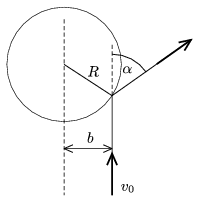(6 pont)

statistics## Problems with sign 'P'

Deadline expired on March 11, 2008.

P. 4043. A ball of weight 2 N can be kept under the water with a force of 8 N. What percent of the volume of the ball is not in the water when the ball is floating on the surface of water?

(3 pont)

statistics

P. 4044. Due to the bad sealing there is some rainwater between the two sheets of the glass window of a train. The water formed a layer of height 2--3 cm. Observing the position of the water layer, what kind of conclusions can be deduced about the motion of the train?

(3 pont)

statistics

P. 4045. Two resistors of resistances 600 ohms and 400 ohms are connected in series and they are connected to a 90 V voltage supply. The voltmeter connected across the 600 ohm resistor reads 45 V. What is the resistance of the voltmeter?

(4 pont)

statistics

P. 4046. The figure shows a smooth vertical path. On the left-handside there is a ramp, the incline angle is=45o, than it is continued with a semi-circle of radius R, and then there is a parabola at the end. The symmetry axis of the parabola is vertical, and the parabola is open above. If a body travel through the path without friction, the normal force exerted on it does not change abruptly at the border of the semicircle and the parabola.

a) What is the height h of the parabola?

b) What is the least height H, measured from the ground, at which an object should be released in order to go through the whole path?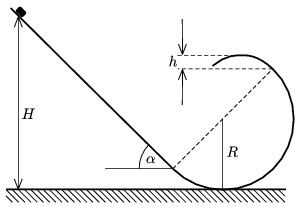(5 pont)

statistics

P. 4047. A uniform density rod of mass m and of lengthis standing vertically on a frictionless surface. The rod is moved out of its unstable equilibrium position.

a) What will be the speeds of the two ends of the rod when it reaches the ground?

b) What is the kinetic energy of the rod at this instant?

(5 pont)

statistics

P. 4048. Inside a spherical container of mass M=385 kg and of cross-section A=1.6 m2 there is a hanging weight, which can slide easily, above a spring, winded round a thin stick. The mass of the weight is m=1.5 kg, and the spring constant is D=155 N/m. (At the beginning the spring is unstretched.) The container is falling from a weather balloon, which is at high altitude, without initial speed. At the beginning of the fall the hanging of the weight is ceased. At what distance from the bottom of the weight should the electric sensor be placed in order to switch on the device which opens the parachute when the container reaches a speed of v=80 m/s? Assume that the density of air is constant.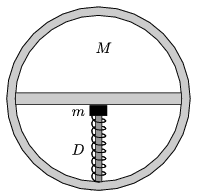(5 pont)

statistics

P. 4049. A sample of diatomic gas of certain mass is taken from state A to state B as shown in the figure; p0=105 Pa, V0=2 dm3.

a) Determine the pressure and the volume of the gas at the intermediate state C until which only QAC=3150 J heat was added to the gas.

b) By what factor does the internal energy of the gas changed during process AC?

c) How much work was done by the expanding gas during process AC?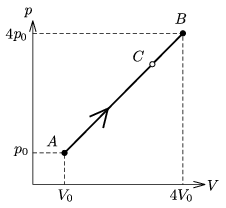(4 pont)

statistics

P. 4050. One of the sides of the frame shown in the figure can move. The frame is in uniform magnetic field of, which is perpendicular to the plane of the frame. At the time of t=0 the magnetic field begins to decrease as.

With what speed should the side of the frame be moved in order not to induce electric current in it?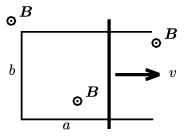(4 pont)

statistics

P. 4051. Draw the image of the segment, shown in the figure, formed by the thin converging lens.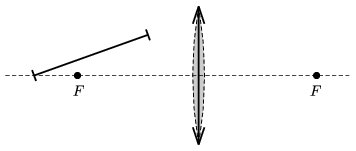(5 pont)

statistics

P. 4052. The window of a room is covered by black paper. There is a square-shaped hole on the paper, through which the red light of the setting sun - nearly perpendicularly - is projected onto the opposite white wall. The distance between the wall and the window is 3.2 m. What is the size and the shape of the light spot on the wall if the area of the square is

a) 1 dm2;

b) 1 mm2;

c) 10-10 m2?

(5 pont)

statistics